# Venmani A D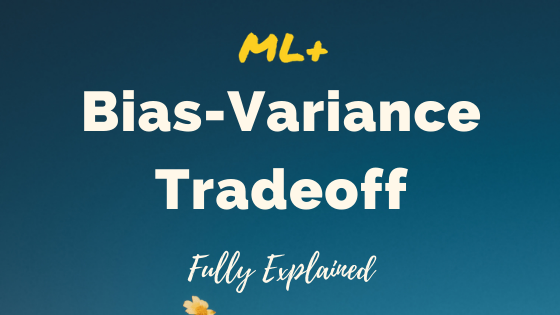## Bias Variance Tradeoff – Clearly Explained

Bias Variance Tradeoff is a design consideration when training the machine learning model. Certain algorithms inherently have a high bias and low variance and vice-versa. In this one, the concept of bias-variance tradeoff is clearly explained so you make an informed decision when training your ML models Bias Variance Tradeoff – Clearly Explained. Photo by …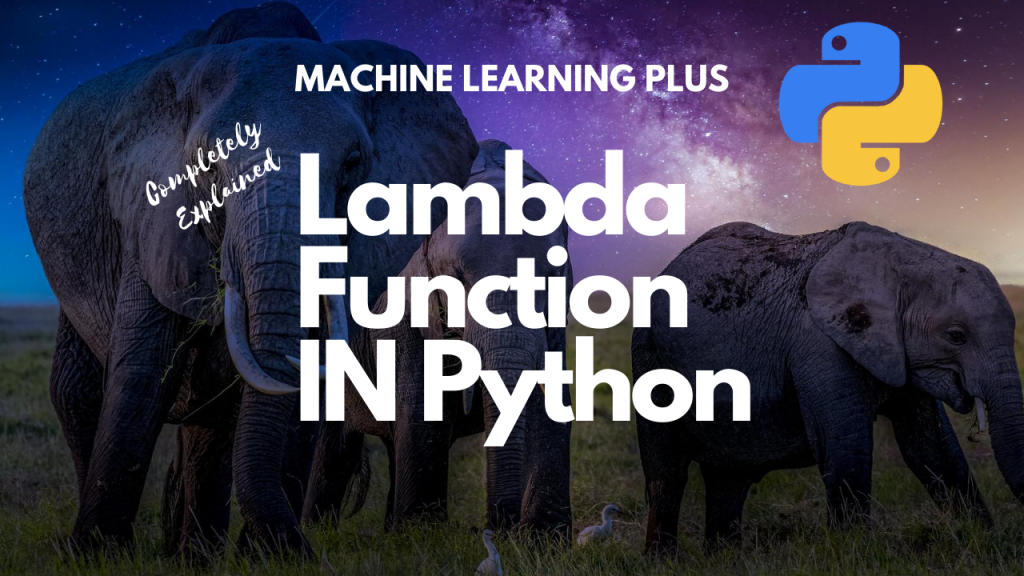## Lambda Function in Python – How and When to use?

Lambda Function, also referred to as ‘Anonymous function’ is same as a regular python function but can be defined without a name. 1. What is Lambda Function in Python? Lambda Function, also referred to as ‘Anonymous function’ is same as a regular python function but can be defined without a name. While normal functions are …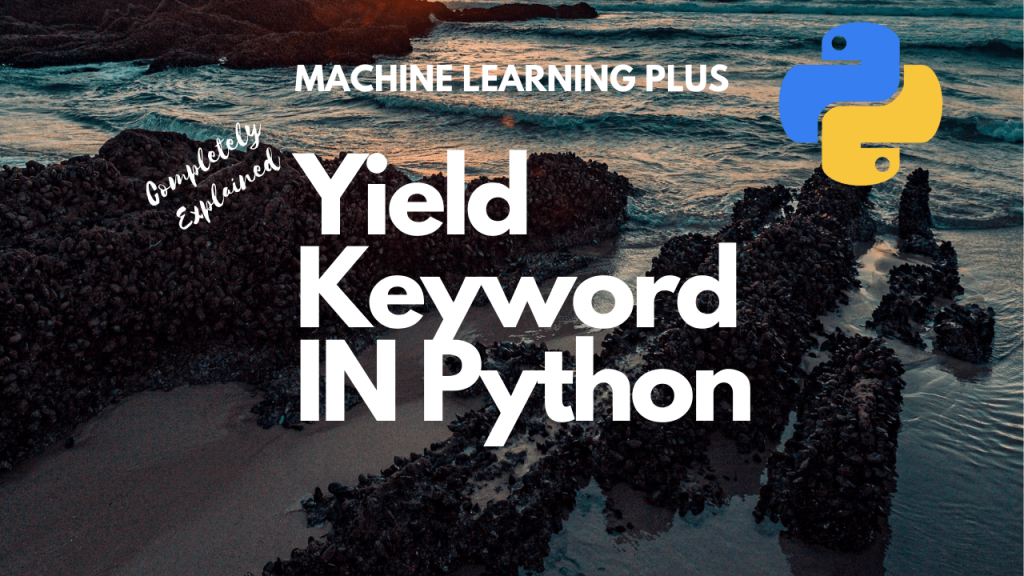## Python Yield – What does the yield keyword do?

Adding yield keyword to a function will make the function return a generator object that can be iterated upon. What does the yield keyword do? Approaches to overcome generator exhaustion How to materialize Generators? How yield works, step by step Exercise 1: Write a program to create a generator that generates cubes of numbers up …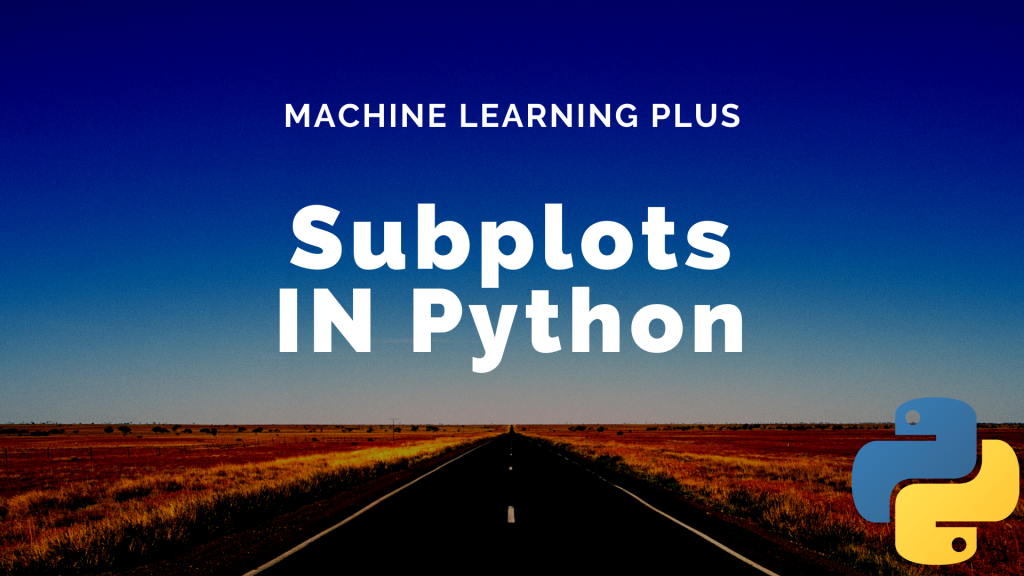## Subplots Python (Matplotlib)

Subplots mean groups of axes that can exist in a single matplotlib figure. subplots() function in the matplotlib library, helps in creating multiple layouts of subplots. It provides control over all the individual plots that are created. Matplotlib Subplots in Python CONTENTS Basic Overview axes() function add_axis() function Creating multiple grids in the same graph …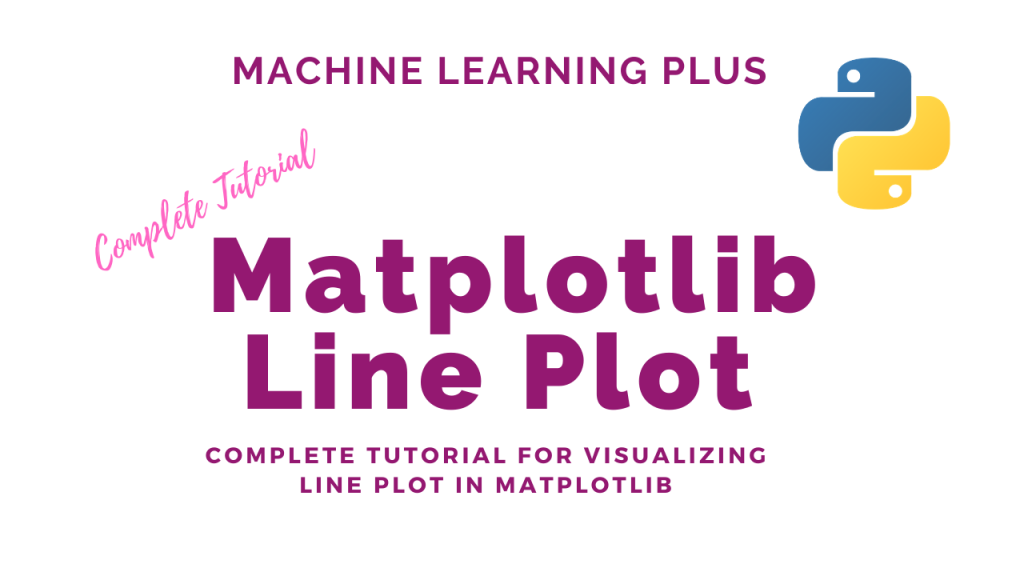## Matplotlib Line Plot

Line plot is a type of chart that displays information as a series of data points connected by straight line segments. A line plot is often the first plot of choice to visualize any time series data. Contents What is line plot? Simple Line Plot Multiple Line Plot in the same graph Creating a secondary …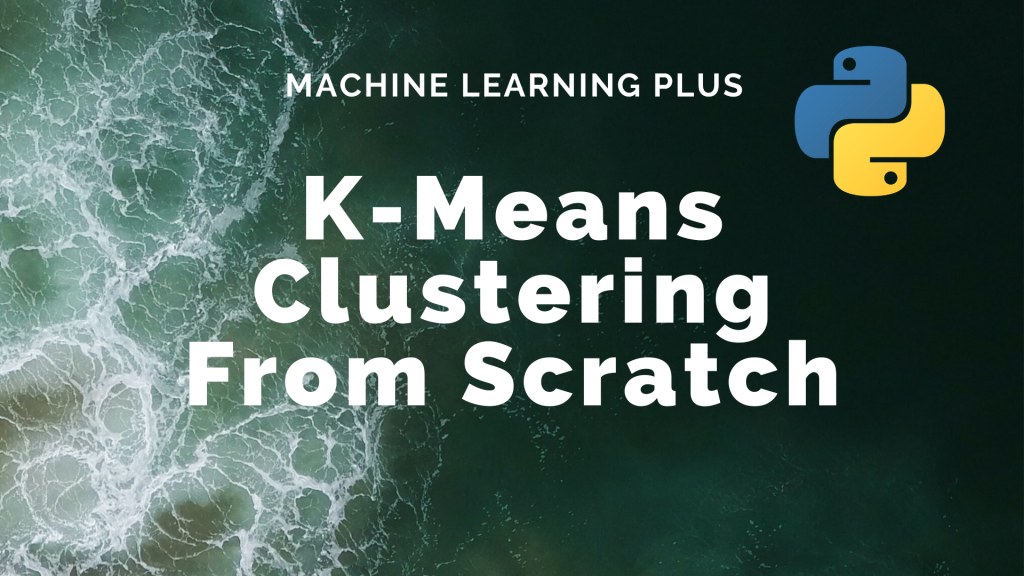## K-Means Clustering Algorithm from Scratch

K-Means Clustering is an unsupervised learning algorithm that aims to group the observations in a given dataset into clusters. The number of clusters is provided as an input. It forms the clusters by minimizing the sum of the distance of points from their respective cluster centroids. Contents Basic Overview Introduction to K-Means Clustering Steps Involved …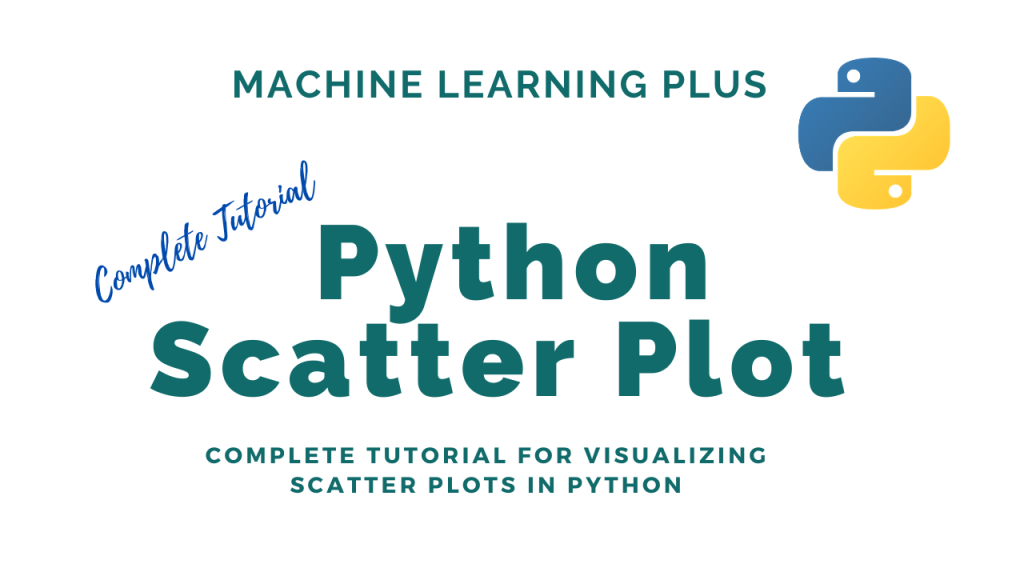## Python Scatter Plot

Scatter plot is a graph in which the values of two variables are plotted along two axes. It is a most basic type of plot that helps you visualize the relationship between two variables. Concept What is a Scatter plot? Basic Scatter plot in python Correlation with Scatter plot Changing the color of groups of …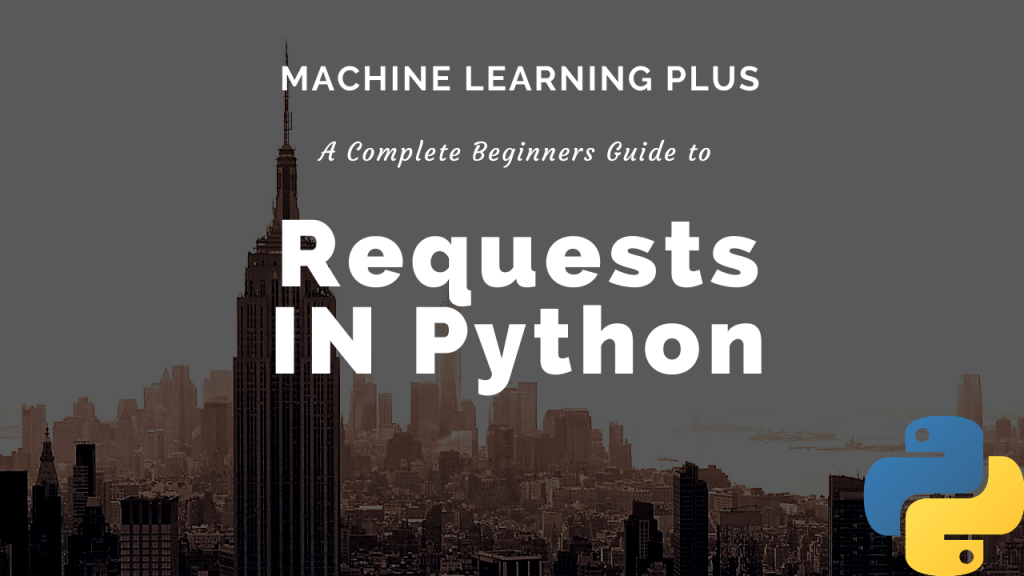## Requests in Python (Guide)

Requests is an elegant and simple Python library built to handle HTTP requests in python easily. It allows you make GET, POST, PUT and other types of requests and process the received response in a flexible Pythonic way. Contents Introduction to Requests Library What is a GET and POST request? GET Method Status Code Contents …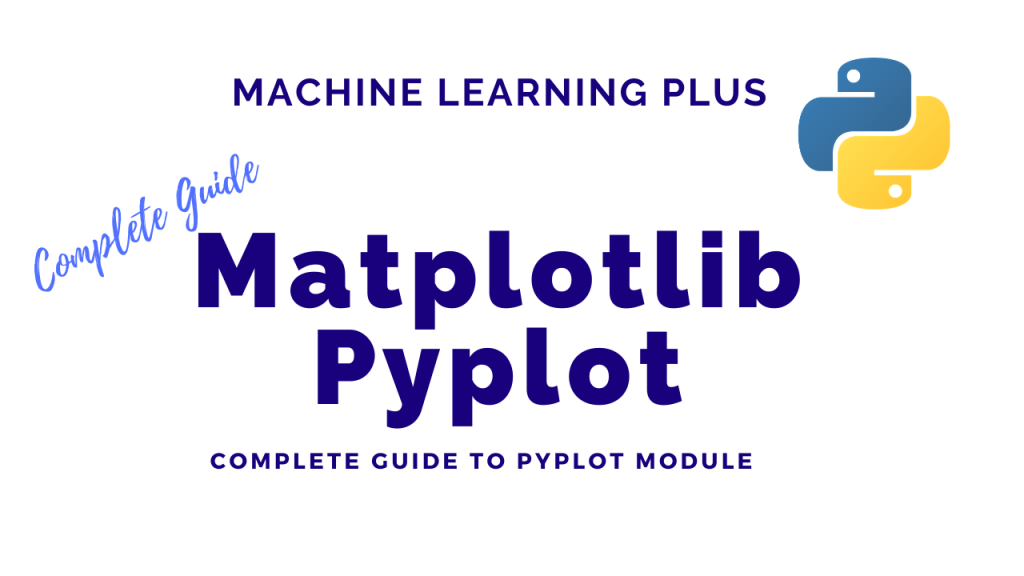## Matplotlib Pyplot

The pyplot object is the main workhorse of matplotlib library. It is through pyplot that you can create the figure canvas, various types of plots, modify and decorate them. Contents Pyplot: Basic Overview General Functions in pyplot Line plot Scatter plot Pie chart Histogram 2D Histograms Bar plot Stacked Barplot Boxplot Stackplot Time series plotting …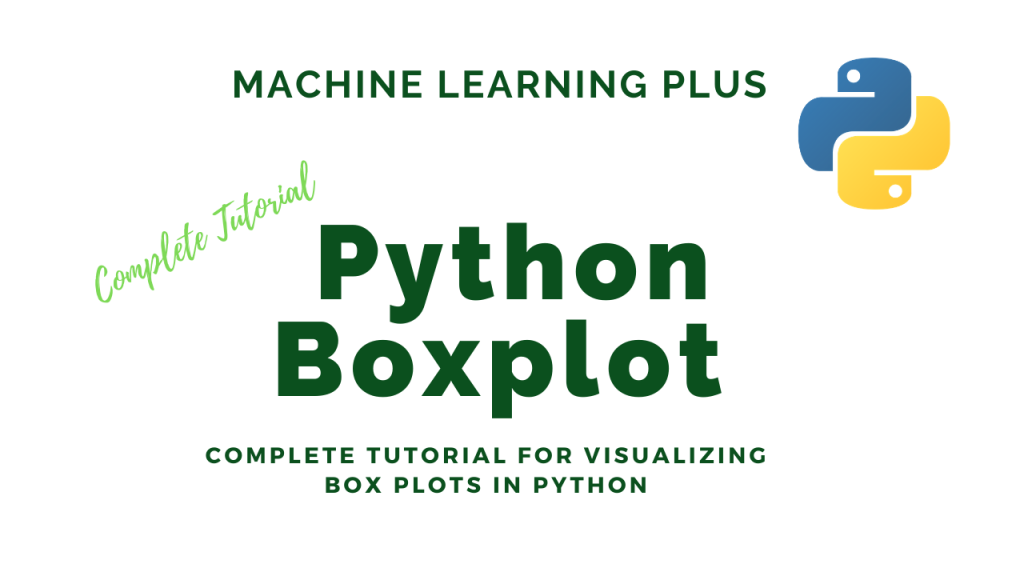## Python Boxplot

Boxplot is a chart that is used to visualize how a given data (variable) is distributed using quartiles. It shows the minimum, maximum, median, first quartile and third quartile in the data set. What is a boxplot? Box plot is method to graphically show the spread of a numerical variable through quartiles. From the below …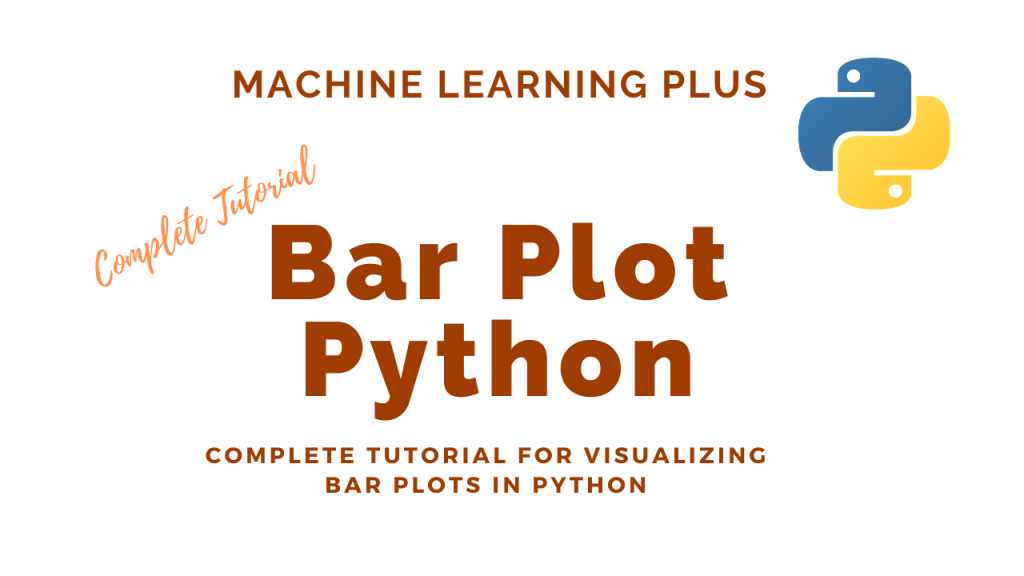## Bar Plot in Python

A bar plot shows catergorical data as rectangular bars with the height of bars proportional to the value they represent. It is often used to compare between values of different categories in the data. Content What is a barplot? Simple bar plot using matplotlib Horizontal barplot Changing color of a barplot Grouped and Stacked Barplots …Course Preview

## Machine Learning A-Z™: Hands-On Python & R In Data Science

### Free Sample Videos:#### Machine Learning A-Z™: Hands-On Python & R In Data Science#### Machine Learning A-Z™: Hands-On Python & R In Data Science#### Machine Learning A-Z™: Hands-On Python & R In Data Science#### Machine Learning A-Z™: Hands-On Python & R In Data Science